Question
Use Nodal analysis to find V( magnitude and angle ). V is in top right.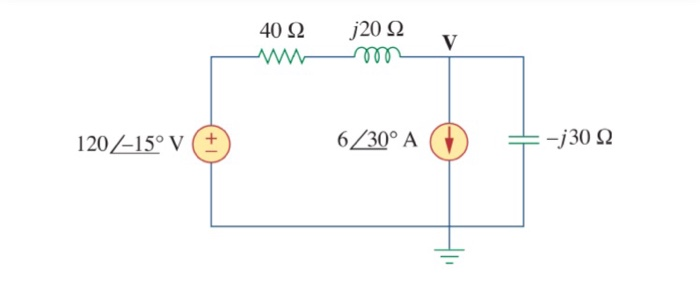solution: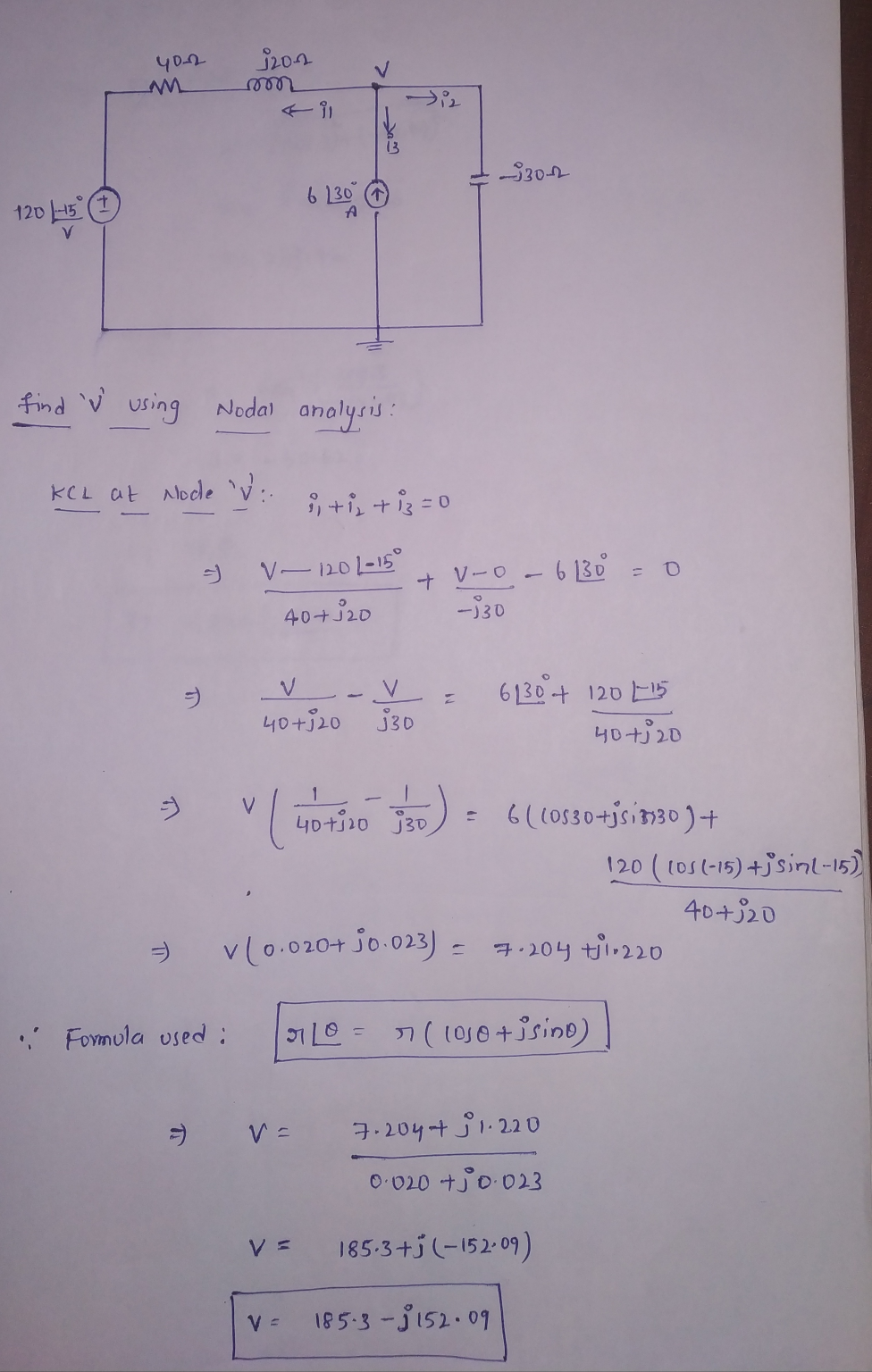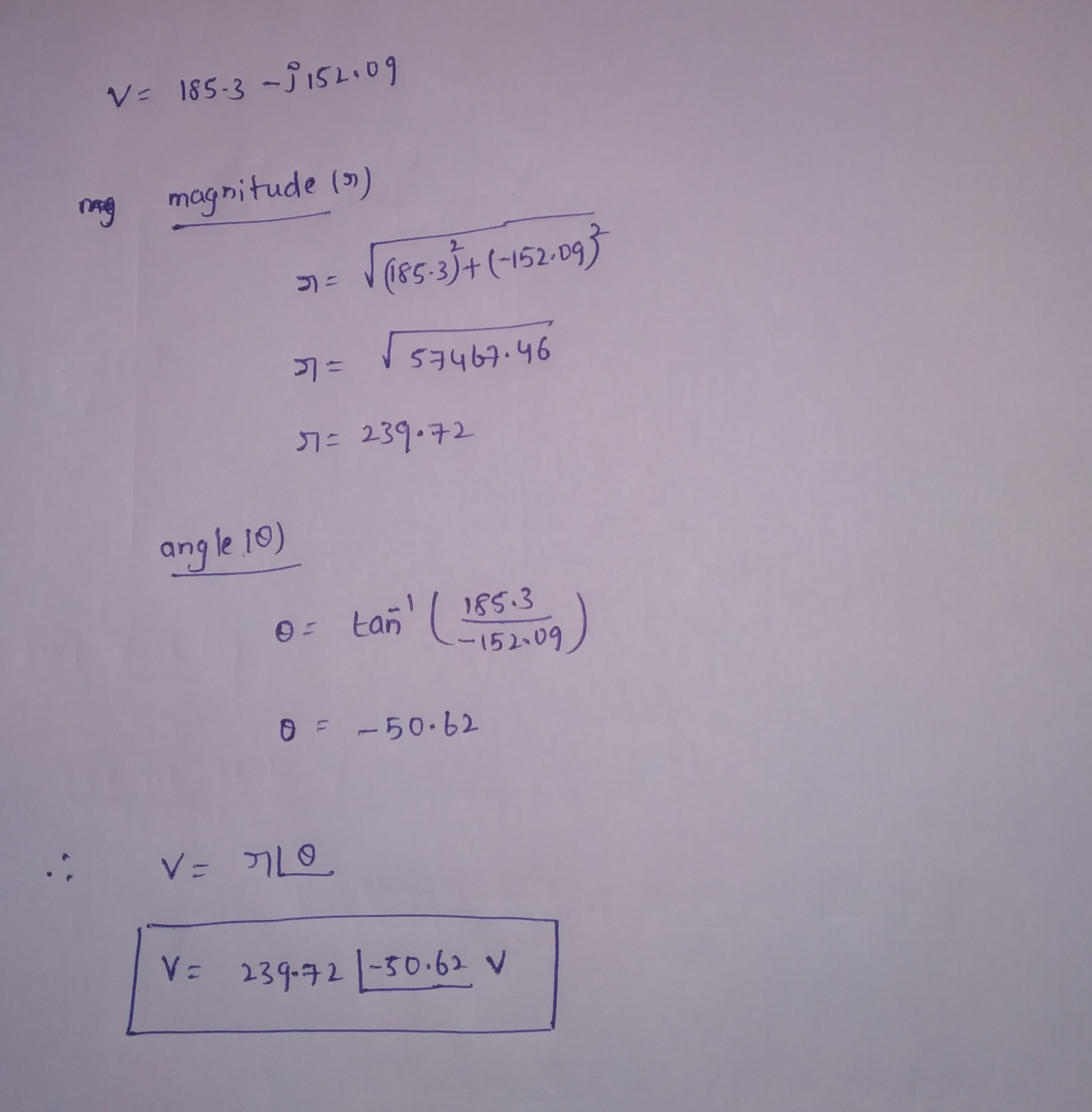I hope the answer is clear. Thank you.

#### Earn Coins

Coins can be redeemed for fabulous gifts.

Similar Homework Help Questions
• ### Find the phasor voltage V using nodal analysis. R = 1812 © = 33° v j2022...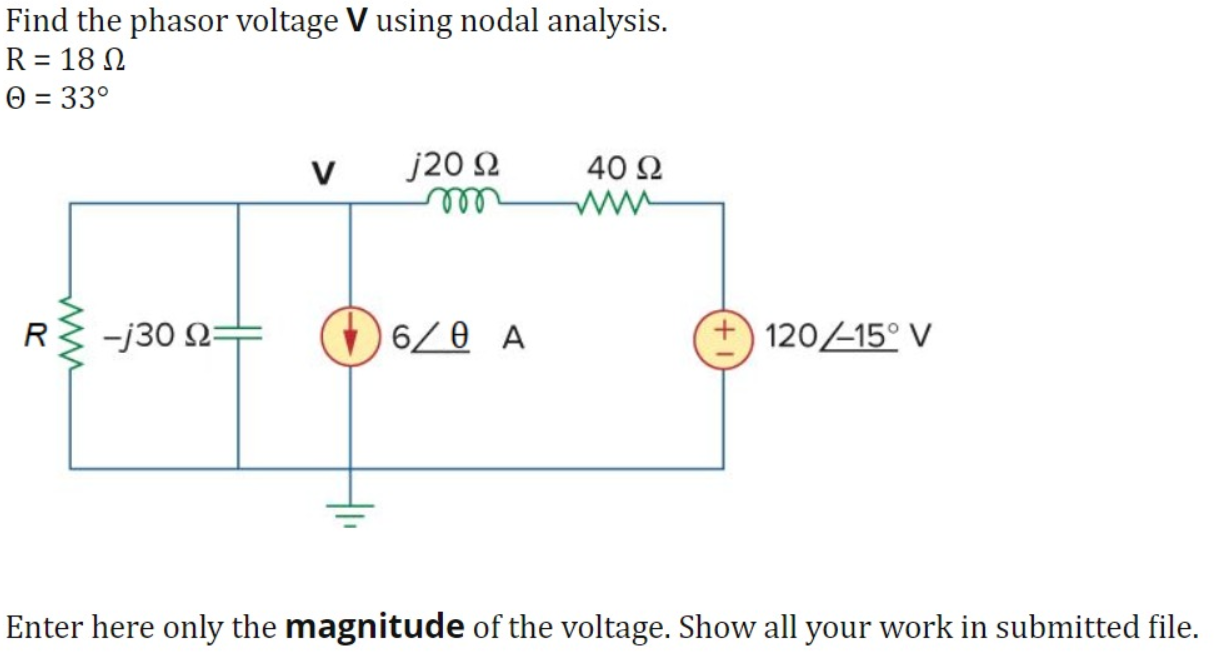Find the phasor voltage V using nodal analysis. R = 1812 © = 33° v j2022 400 R} -j30 + 60 A 120/-15° V Enter here only the magnitude of the voltage. Show all your work in submitted file.

• ### Use voltage rise and voltage drop with respect to a clockwise loop. Nodal analysis In Exercises...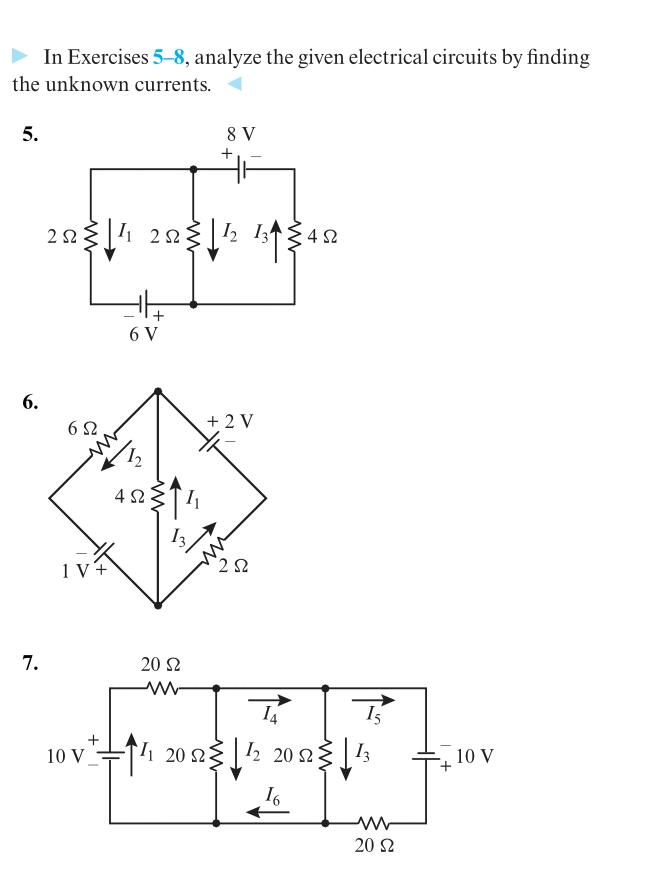Use voltage rise and voltage drop with respect to a clockwise loop. Nodal analysis In Exercises 5-8, analyze the given electrical circuits by finding the unknown currents. 5. 8V + 2 Ωs |Ι 2 Ωξ | Ι, 15 4Ω + 6V 6. 6Ω + 2V 4 Ω 1V + 2Ω 7. 20 Ω Ι4 15 10 V 20 Ω 12 20 Ω 13 10 V 20 Ω

• ### Transform the circuit below into the frequency domain, then use nodal analysis to find V(ω), the...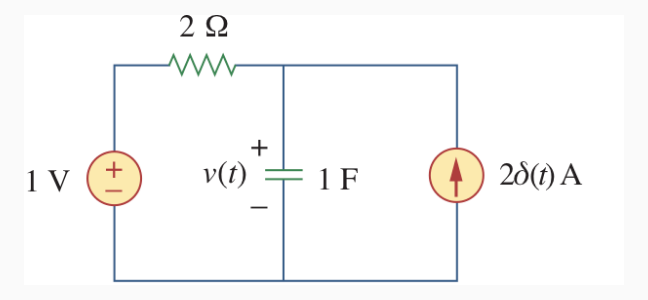Transform the circuit below into the frequency domain, then use nodal analysis to find V(ω), the Fourier transform of v(t). 212 www + + 1 V v(t) 1F 28(t) A

• ### Just wanna check if its right? Shin 3 2 (20 pts) Use nodal analysis to find...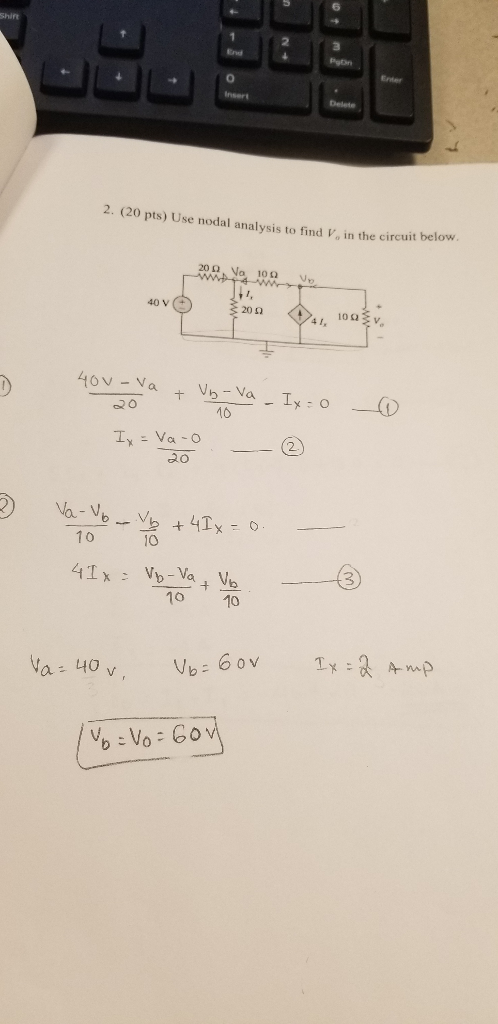Just wanna check if its right? Shin 3 2 (20 pts) Use nodal analysis to find V, in the circuit belotw 40 V ( 20Ω 16 2 ao 0 10 10 10 60v A- n a-40 v 醫. : 60

• ### Using Nodal analysis to find lo 3νο 3 Ω 6 Ω 6 Ω 10 V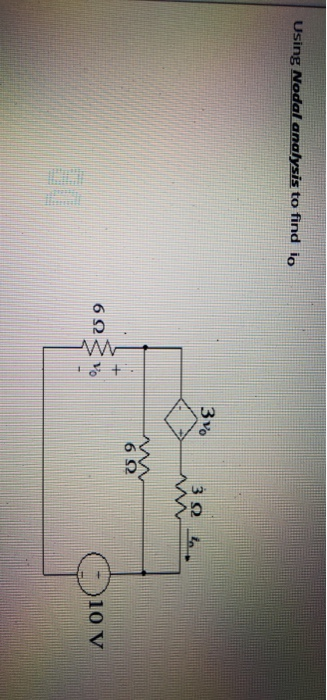Using Nodal analysis to find lo 3νο 3 Ω 6 Ω 6 Ω 10 V

• ### Use nodal analysis to find V in the circuit of Figure Q5.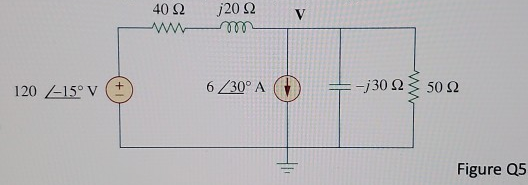Use nodal analysis to find V in the circuit of Figure Q5.

• ### 8. Use Nodal Analysis to solve for V 10V 411 40 12V 60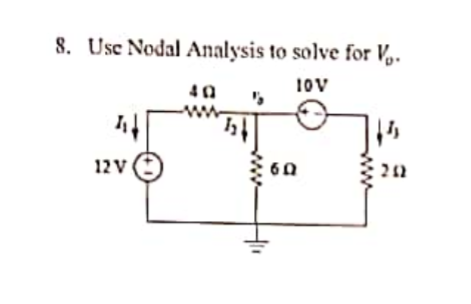8. Use Nodal Analysis to solve for V 10V 411 40 12V 60

• ### 3-1 Use nodal analysis to find v, in the circuit shown in Fig. 3-6 if element...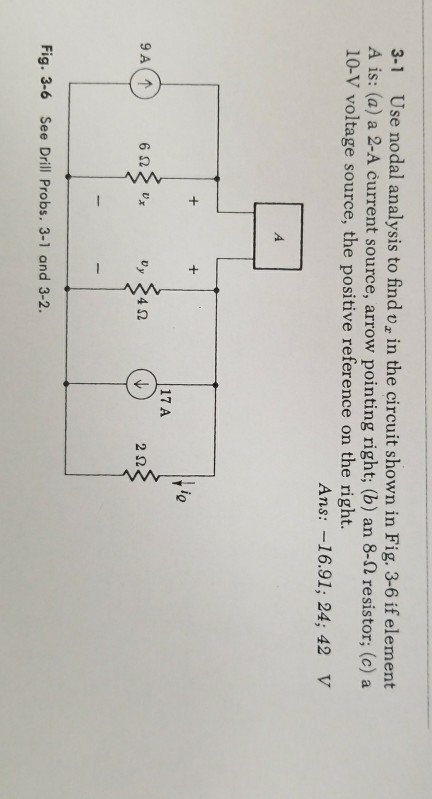3-1 Use nodal analysis to find v, in the circuit shown in Fig. 3-6 if element A is: (a) a 2-A current source, arrow pointing right; (b) an 8-92 resistor; (c) a 10-V voltage source, the positive reference on the right. ns:- 17 A 2Ω Fig. 3-6 See Drill Probs. 3-1 and 3-2.

• ### 3-6: Use nodal analysis to find the values of i1, i2, and iz: 1 kΩ 10...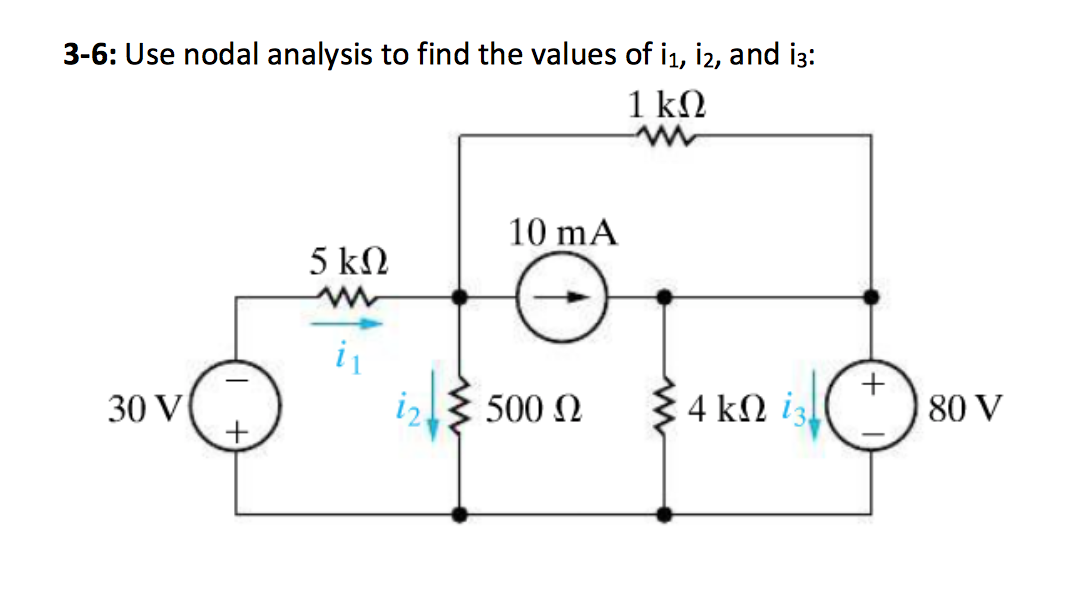3-6: Use nodal analysis to find the values of i1, i2, and iz: 1 kΩ 10 mA 5 kΩ 11 30v) 1 ξ 500 Ω ξ4 ΚΩ (Ε) 80 V

• ### 7. Use nodal analysis to solve for the nodal voltages in the following circuit: 2Α (1)...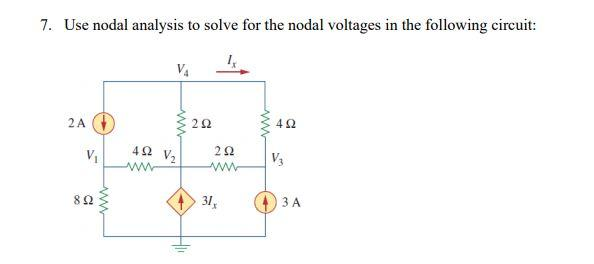7. Use nodal analysis to solve for the nodal voltages in the following circuit: 2Α (1) WW 2Ω Μν 4Ω V V 4 Ω V. 2Ω W ΑΛΛ 8Ω ΑΛ 31, 3Α Η